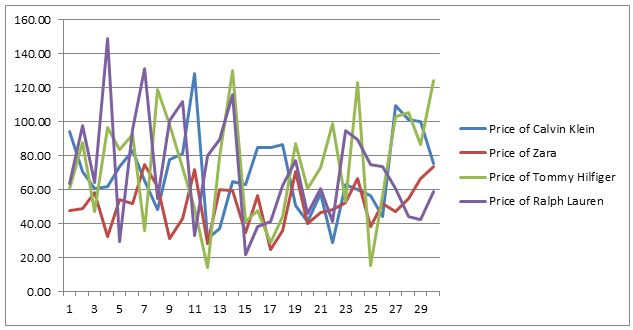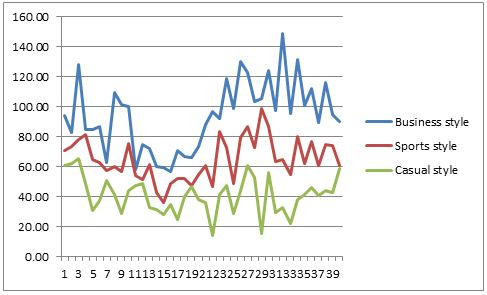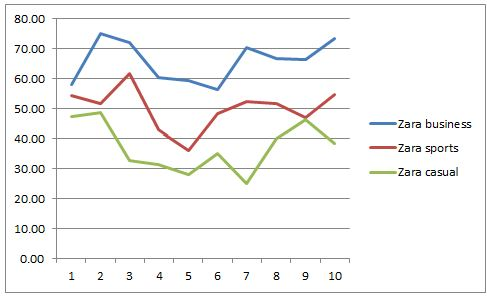Essentials of Statistics and Economics Proof Reading ServicesQuestion 1

Visual presentation of the prices based on product from different brands

 Price of Calvin Klein Price of Zara Price of Tommy Hilfiger Price of Ralph Lauren 94.27 47.53 60.73 63.77 70.71 48.87 87.92 97.55 60.48 58.19 47.07 64.47 61.83 32.64 96.78 149.02 73.78 54.35 83.47 29.53 82.79 51.75 92.30 95.28 65.26 75.09 35.89 131.19 48.48 61.67 118.92 54.59 78.00 31.28 98.56 100.66 81.44 42.92 72.89 111.94 128.17 72.02 49.06 32.78 31.00 28.04 14.38 80.25 37.42 60.34 79.39 89.56 64.86 59.32 130.20 115.84 63.02 34.98 41.18 22.08 84.65 56.49 47.57 38.35 84.74 25.07 29.02 41.41 86.82 36.22 44.24 62.18 50.59 70.48 87.10 77.11 41.17 39.91 60.49 45.88 57.74 46.55 72.93 60.77 29.09 48.50 98.93 41.05 63.03 52.36 52.82 94.84 60.27 66.86 123.02 89.81 56.75 38.36 15.42 74.94 44.24 51.88 56.38 73.80 109.63 47.16 103.36 60.55 101.15 54.65 105.25 44.30 100.12 66.34 86.78 42.48 75.55 73.45 124.03 59.07

ChartsOne of the fundamental aspects in representing the data output on prices is through distribution. The price distribution arises because the rates will not yield the same at every time due to various macro and micro economic factors.  Therefore, there exist a scattering of values around central tendency. The location, shape and variability of the distributions can be analysed using the following tools. (Freedman, 2010)

Location – Mean & median; Spread – Variance, Standard deviation and range and Shape – Skewness and Kurtosis

The descriptive statistics of prices

 Calvin Klein Location Mean 69.56889 Median 65.05888 Spread Standard Deviation 23.37837 Sample Variance 546.5483 Range 99.08802 Shape Kurtosis 0.111549 Skewness 0.401199 Minimum 29.08674 Maximum 128.1748 Count 30
 Zara Location Mean 51.10847 Median 51.8144 Spread Standard Deviation 13.86985 Sample Variance 192.3726 Range 50.02136 Shape Kurtosis -0.77666 Skewness -0.05338 Minimum 25.06978 Maximum 75.09114 Count 30
 Tommy Hilfiger Location Mean 73.86924 Median 76.16283 Spread Standard Deviation 32.29041 Sample Variance 1042.671 Range 115.8186 Shape Kurtosis -0.86718 Skewness -0.06482 Minimum 14.38371 Maximum 130.2023 Count 30
 Ralph Lauren Location Mean 71.50168 Median 64.12269 Spread Standard Deviation 31.26372 Sample Variance 977.4204 Range 126.9439 Shape Kurtosis -0.07787 Skewness 0.600018 Minimum 22.07529 Maximum 149.0192 Count 30

From the above descriptive analysis it is noted that the mean and median value of Calvin Klein is different, the mean states the average of the distribution whereas the median states the 50 percentile of the data or the mid value. However, it is noted that mean and median of Zara is almost same at 51, however Tommy Hilfiger and Ralph Lauren is also have a difference between the mean and medianWhile measuring the spread of the data, the standard deviation of Calvin Klein, Tommy Hilfiger and Ralph Lauren has highest value, this shows that the deviation of the values from the mean is huge. Therefore a large standard deviation means the observed value has a huge difference when compared with mean. Therefore the goal should be less standard deviation which is noted in Zara. Therefore, it can be stated that the mean value of Zara has more meaningful information . (Triola, 2014)

In order to analyse the shape of the data, skewness and kurtosis is applied, skewness will state the symmetry of the data. A data to be normally distributed should possess the value of skewness as 0. Similarly Kurtosis is used to measure the combined size of two tails of information, for a data to be normally distributed should have value equal to 3. In the descriptive analysis it is noted that Zara and Tommy Hilfiger has a skewness value almost 0, whereas Calvin Klein and Ralph Lauren have values more than 0, therefore these two products are asymmetrical and the prices may not have normal distribution.

Question 2

Visual representationBusiness Style Location Mean 89.14395 Median 87.36749 Spread Standard Deviation 22.28638 Sample Variance 496.6829 Range 73.71693 Shape Kurtosis -0.94622 Skewness 0.274778 Minimum 56.48535 Maximum 130.2023 Count 30
 Sports style Location Mean 64.06367 Median 61.19693 Spread Standard Deviation 15.32209 Sample Variance 234.7665 Range 62.71523 Shape Kurtosis -0.6026 Skewness 0.34497 Minimum 36.21763 Maximum 98.93286 Count 30
 Casual style Location Mean 41.33898 Median 41.17424 Spread Standard Deviation 13.02517 Sample Variance 169.655 Range 50.87537 Shape Kurtosis -0.36824 Skewness -0.11966 Minimum 14.38371 Maximum 65.25908 Count 30

From the above descriptive analysis it is noted that the mean and median value of Business style and Sports style wears are different, the mean states the average of the distribution whereas the median states the 50 percentile of the data or the mid value. However, it is noted that mean and median of Casual style wear is almost same at 41. Therefore the mean and median is closely related for Casual style wear. (Boslaugh, 2012)

While measuring the spread of the data, the standard deviation of Business style has highest value with 22.28, this shows that the deviation of the values from the mean is huge. Therefore a large standard deviation means the observed value has a huge difference when compared with mean. Therefore the goal should be less standard deviation which is noted in Sports style and Casual style with SD of 15.32 and 13.02 respectively. Therefore, it can be stated that the mean value of Business style and Casual style has more meaningful information .

In order to analyse the shape of the data, skewness and kurtosis is applied, skewness will state the symmetry of the data. A data to be normally distributed should possess the value of skewness as 0. Similarly Kurtosis is used to measure the combined size of two tails of information, for a data to be normally distributed should have value equal to 3. In the descriptive analysis it is noted that Casual style has a skewness value almost 0, whereas Business style and Sports style have values more than 0, therefore these two style are asymmetrical and the prices may not have normal distribution.

Question 3

 Zara business Zara sports Zara casual 58.19 54.35 47.53 75.09 51.75 48.87 72.02 61.67 32.64 60.34 42.92 31.28 59.32 36.22 28.04 56.49 48.50 34.98 70.48 52.36 25.07 66.86 51.88 39.91 66.34 47.16 46.55 73.45 54.65 38.36Zara business style Location Mean 65.85872 Median 66.60369 Spread Standard Deviation 6.859775 Sample Variance 47.05652 Range 18.60579 Shape Kurtosis -1.73364 Skewness -0.07044 Minimum 56.48535 Maximum 75.09114 Count 10
 Zara sports style Location Mean 50.14484 Median 51.8144 Spread Standard Deviation 6.984534 Sample Variance 48.78372 Range 25.45067 Shape Kurtosis 1.056389 Skewness -0.56811 Minimum 36.21763 Maximum 61.6683 Count 10
 Zara casual style Location Mean 37.32185 Median 36.6685 Spread Standard Deviation 8.370247 Sample Variance 70.06104 Range 23.8008 Shape Kurtosis -1.32792 Skewness 0.102239 Minimum 25.06978 Maximum 48.87058 Count 10

From the above descriptive analysis it is noted that the mean and median value of all style of products of Zara looks same, the mean states the average of the distribution whereas the median states the 50 percentile of the data or the mid value. However, it is noted that mean and median of all products of Zara  is almost same.

The goal should be less standard deviation which is noted in Business style, Sports style and Casual style of Zara products are less. Therefore, it can be stated that the mean value of all products  are closely related to the mean value.

In order to analyse the shape of the data, skewness and kurtosis is applied, skewness will state the symmetry of the data. A data to be normally distributed should possess the value of skewness as 0. Similarly Kurtosis is used to measure the combined size of two tails of information, for a data to be normally distributed should have value equal to 3. In the descriptive analysis it is noted that business style has a skewness value almost 0, whereas sports style and Sports style have values more than 0, therefore these two style are asymmetrical and the prices may not have normal distribution. (Witte, 2010)

Question 4

A t-test is one of the statistical test which helps the researcher to determine whether the two set of data is significantly different from each other. A t test is one of the commonly implemented test analysis, which will follow the steps of the normal distribution. It should be noted that when the scaling term is unknown and it is replaced by an estimate which is available on the data then we can follow students t-test. (Sincich, 2012)

The first step is to formulate the hypothesis, the null hypothesis will state that there mean difference is the same or to put it in other words, there is no significant difference between the variables. The alternate hypothesis will be stated as there is a significant difference among the variables. The interpretation of the data can be stated if the test statistic value is greater than the significance value then null hypothesis is rejected and alternate hypothesis is accepted. (Freedman, 2010)

In the given problem, the objective is to test if there is a significant difference in average prices across these two gender targets. Therefore the following hypothesis is framed.

Null hypothesis: There is no significant difference between average prices and gender

Alternate hypothesis:There is a significant difference between average prices and gender

 t-Test: Two-Sample Assuming Unequal Variances Price Gender Mean 66.51207 1.5 Variance 754.4582 0.252101 Observations 120 120 Hypothesized Mean Difference 0 df 119 t Stat 25.92355 P(T<=t) one-tail 0.004 t Critical one-tail 1.657759 P(T<=t) two-tail 0.008 t Critical two-tail 1.9801

From the above t-test analysis it can be noted that mean value of price is 66.51 and the gender is 1.5, the variance of prices are high when compared with the gender.

It is further noted that t statistic is 25.92 whereas the p value is 0.004, this shows that the mean of values between price changes and gender are same. Therefore, null hypothesis is accepted. Hence it is stated that there is no significant difference between average prices and gender.

Question 5

A t-test is one of the statistical test which helps the researcher to determine whether the two set of data is significantly different from each other. A t test is one of the commonly implemented test analysis, which will follow the steps of the normal distribution. It should be noted that when the scaling term is unknown and it is replaced by an estimate which is available on the data then we can follow students t-test.

The first step is to formulate the hypothesis, the null hypothesis will state that there mean difference is the same or to put it in other words, there is no significant difference between the variables. The alternate hypothesis will be stated as there is a significant difference among the variables. The interpretation of the data can be stated if the test statistic value is greater than the significance value then null hypothesis is rejected and alternate hypothesis is accepted.

In the given problem, the objective is to test if there is a significant difference in average prices across brands. Therefore the following hypothesis is framed.

Null hypothesis:There is no significant difference between average prices and brands

Alternate hypothesis:There is a significant difference between average prices and brands

 t-Test: Two-Sample Assuming Unequal Variances Price Brands Mean 66.27881803 2.512605042 Variance 754.2678226 1.25195841 Observations 119 119 Hypothesized Mean Difference 0 df 118 t Stat 25.30703038 P(T<=t) one-tail 0.008 t Critical one-tail 1.657869523 P(T<=t) two-tail 0.001 t Critical two-tail 1.980272226

From the above t-test analysis it can be noted that mean value of price is 66.27 and the brands is 1.5, the variance of prices are high when compared with the brands.

It is further noted that t statistic is 25.30 whereas the p value is 0.008, this shows that the mean of values between price changes and brands are same. Therefore, null hypothesis is accepted. Hence it is stated that there is no significant difference between average prices and brands.

Question 6

A t-test is one of the statistical test which helps the researcher to determine whether the two set of data is significantly different from each other. A t test is one of the commonly implemented test analysis, which will follow the steps of the normal distribution. It should be noted that when the scaling term is unknown and it is replaced by an estimate which is available on the data then we can follow students t-test.

The first step is to formulate the hypothesis, the null hypothesis will state that there mean difference is the same or to put it in other words, there is no significant difference between the variables. The alternate hypothesis will be stated as there is a significant difference among the variables. The interpretation of the data can be stated if the test statistic value is greater than the significance value then null hypothesis is rejected and alternate hypothesis is accepted. (Freedman, 2010)

In the given problem, the objective is to test if there is a significant difference in average prices across styles. Therefore the following hypothesis is framed.

Null hypothesis: There is no significant difference between average prices and styles

Alternate hypothesis:There is a significant difference between average prices and styles

 t-Test: Two-Sample Assuming Unequal Variances Prices Styles Mean 66.27881803 2.008403361 Variance 754.2678226 0.66942031 Observations 119 119 Hypothesized Mean Difference 0 df 118 t Stat 25.51697319 P(T<=t) one-tail 0.003 t Critical one-tail 1.657869523 P(T<=t) two-tail 0.007 t Critical two-tail 1.980272226

From the above t-test analysis it can be noted that mean value of price is 66.27 and the styles is 2.0, the variance of prices are high when compared with the styles.

It is further noted that t statistic is 25.5 whereas the p value is 0.003, this shows that the mean of values between price changes and styles are same. Therefore, null hypothesis is accepted. Hence it is stated that there is no significant difference between average prices and styles.

Question 7

A t-test is one of the statistical test which helps the researcher to determine whether the two set of data is significantly different from each other. A t test is one of the commonly implemented test analysis, which will follow the steps of the normal distribution. It should be noted that when the scaling term is unknown and it is replaced by an estimate which is available on the data then we can follow students t-test.

The first step is to formulate the hypothesis, the null hypothesis will state that there mean difference is the same or to put it in other words, there is no significant difference between the variables. The alternate hypothesis will be stated as there is a significant difference among the variables. The interpretation of the data can be stated if the test statistic value is greater than the significance value then null hypothesis is rejected and alternate hypothesis is accepted.

In the given problem, the objective is to test if there is a significant difference in Zara average prices across Zara styles. Therefore the following hypothesis is framed.

Null hypothesis:There is no significant difference between Zara average prices and Zara styles

Alternate hypothesis:There is a significant difference between Zara average prices and Zara styles

Zara prices and styles can be grouped as follows

 PRICE STYLE 47.53 3 48.87 3 58.19 1 32.64 3 54.35 2 51.75 2 75.09 1 61.67 2 31.28 3 42.92 2 72.02 1 28.04 3 60.34 1 59.32 1 34.98 3 56.49 1 25.07 3 36.22 2 70.48 1 39.91 3 46.55 3 48.50 2 52.36 2 66.86 1 38.36 3 51.88 2 47.16 2 54.65 2 66.34 1 73.45 1
 t-Test: Two-Sample Assuming Unequal Variances Zara prices Zara styles Mean 51.23195651 1.965517241 Variance 198.7692613 0.677339901 Observations 29 29 Hypothesized Mean Difference 0 df 28 t Stat 18.78610969 P(T<=t) one-tail 0.0014 t Critical one-tail 1.701130908 P(T<=t) two-tail 0.002 t Critical two-tail 2.048407115

From the above t-test analysis it can be noted that mean value of Zara average price is 51.23 and the styles of Zara is 1.96, the variance of prices are high when compared with the styles.

It is further noted that t statistic is 18.8 whereas the p value is 0.001, this shows that the mean of values between price changes and styles are same. Therefore, null hypothesis is accepted. Hence it is stated that there is no significant difference between Zara average prices and Zara styles.

References

Boslaugh, Sarah (2012). Statistics in a Nutshell. Cengage Publishing

Freedman, David (2010). Statistics. 4th Edition. Cengage Publishing

Sincich, T. Terry (2012). Statistics. 12th Edition.

Triola (2014). Essentials of Statistics. 5th Edition. McGraw Hill

Witte, S. Robert. (2010). Statistics. 5th Edition. McGraw Hill